# MATHEMATICS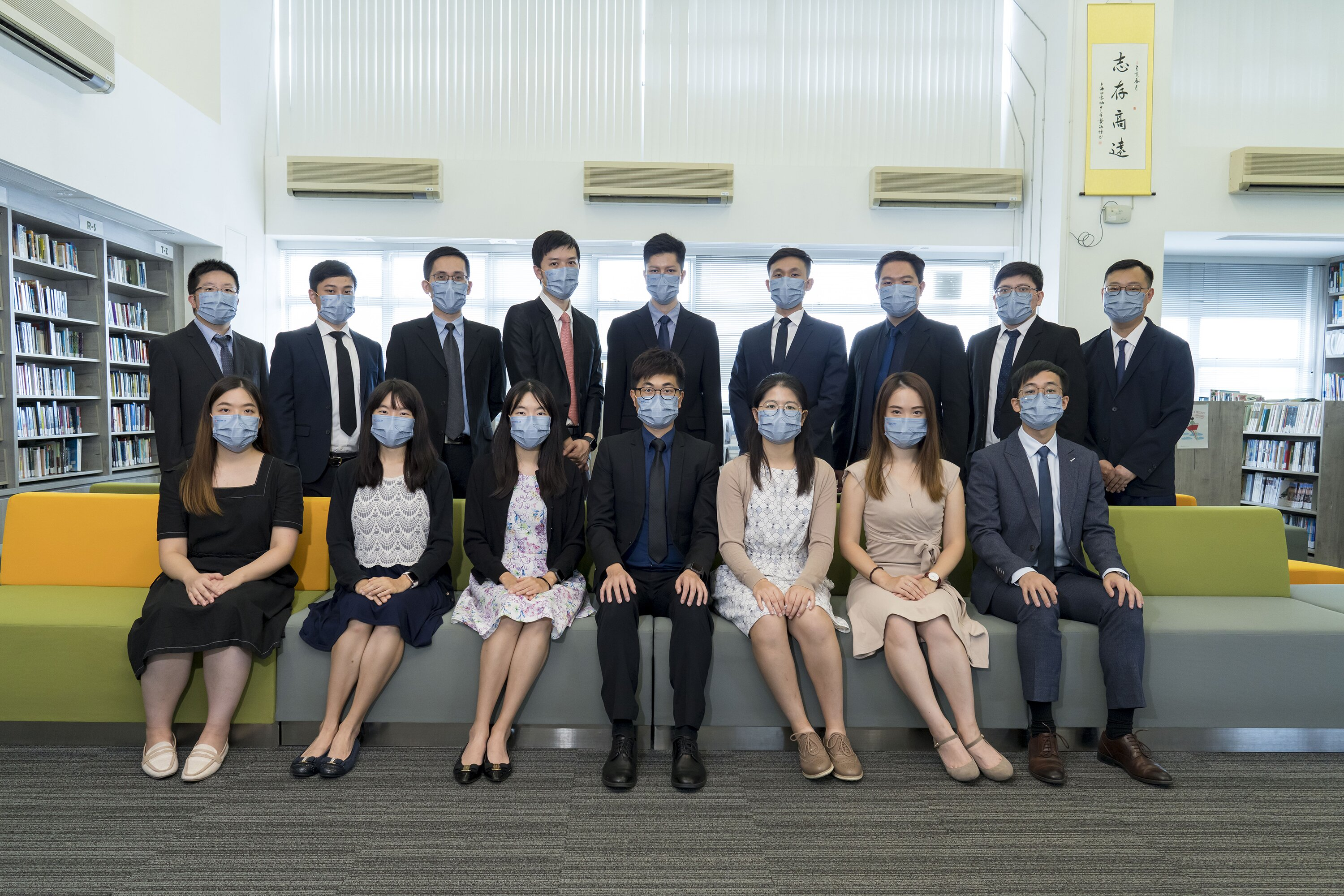The aims of the learning and teaching of mathematics are to enable students to understand concepts and demonstrate skills from different branches of mathematics; to develop the ability to communicate with appropriate symbols and the language of mathematics; to recognize patterns and structures and describe them as relationships or general rules...

Our Members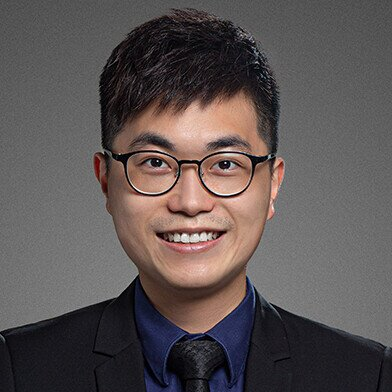Mr. Horace Cheng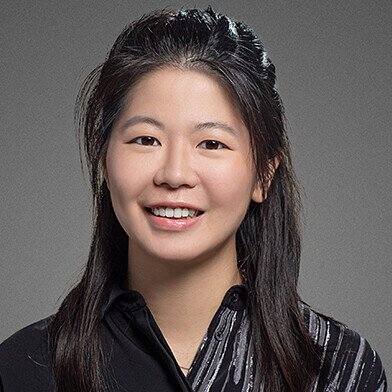Ms. Eunice Li

Mathematics Coordinator (Junior), Mathematics Teacher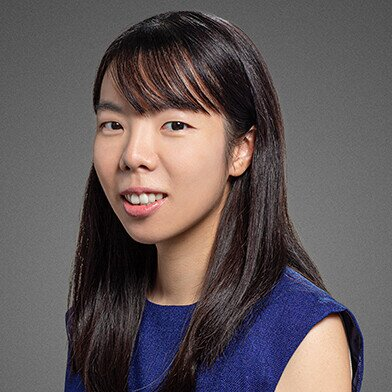Ms. Wing Chan

Mathematics Coordinator (Senior), Mathematics Teacher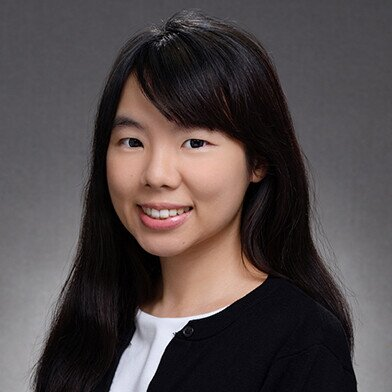Ms. Chong Chan

Mathematics Teacher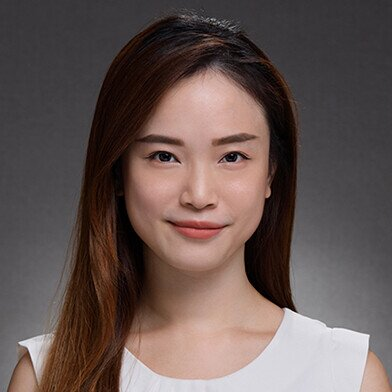Ms. Soying Chan

Mathematics Teacher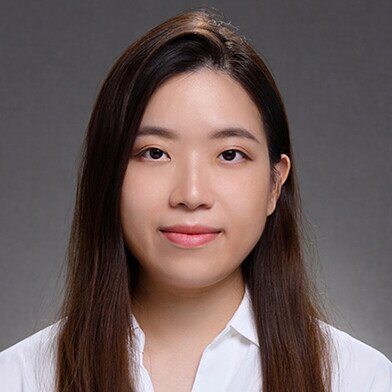Ms. Phoebe Fok

Mathematics Teacher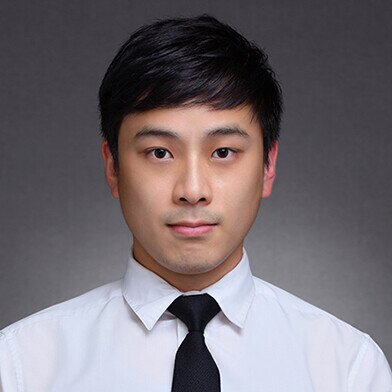Mr. Alan Fong

Mathematics Teacher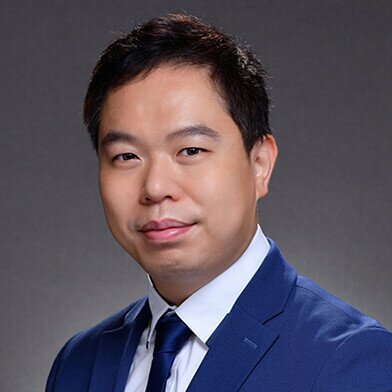Mr. Patrick Lai

Mathematics Teacher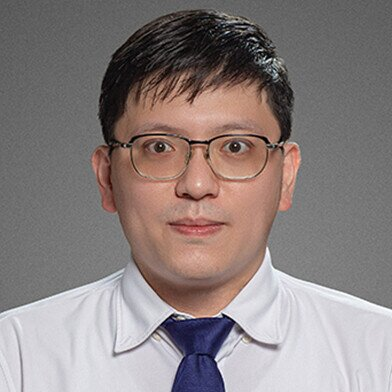Mr. Jimmy Leung

Mathematics Teacher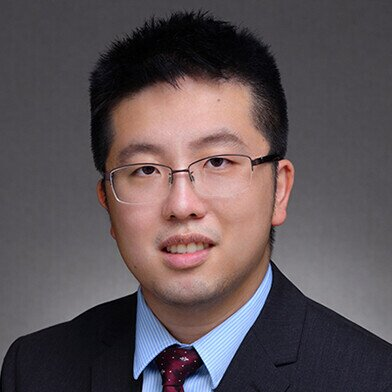Mr. Tom Leung

Mathematics Teacher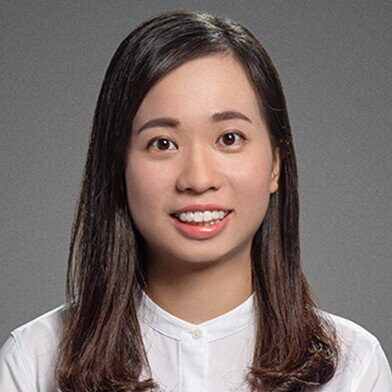Ms. Emily Li

Mathematics Teacher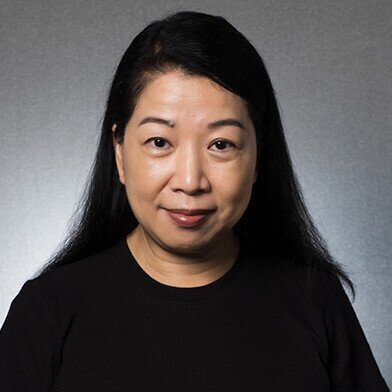Ms. Lilly Ng

Mathematics TeacherMr. Woody Tse

Mathematics TeacherMr. Arthur Yeung

Mathematics Teacher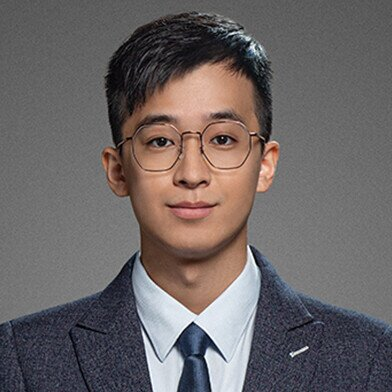Mr. Ricky Leung

Mathematics Teacher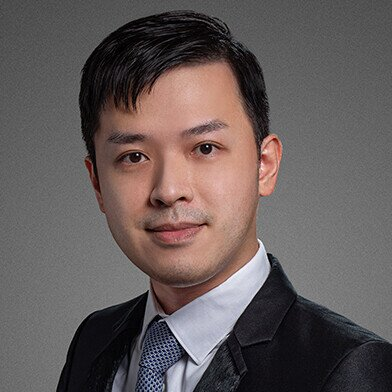Mr. Jonathan Yu

Mathematics Teacher